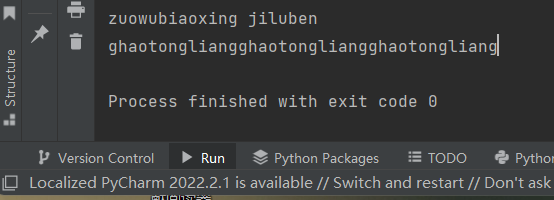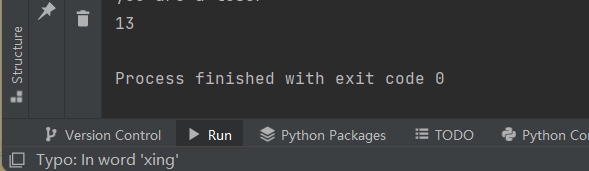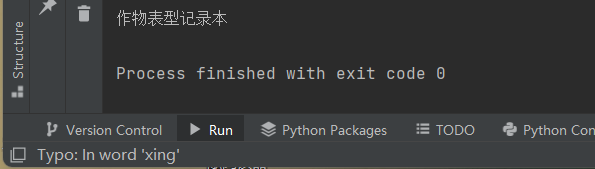1

17633788835

V1

2022/10/14阅读：16主题：全栈蓝

# 运算符

1. 算术运算符和比较运算符： 1.1 算术运算符：
• '+':加法运算符
• '-':减法运算符/负号
• '*':乘法运算符
• '/'：除法运算符
• '**'：幂运算符（计算某一个数的某次方）
• '//'：取整除运算符
• '%'：取模运算符（常用于计算两个正整数相除的余数） '+'和'*'除了能作为算术运算符对数字进行计算，还能作为字符串运算符对字符串进行运算；'+'用于拼接字符串；' * '用于将字符串复制指定的分数：
``a='zuowubiaoxing'b='jiluben'c=a +' '+ bprint(c)d='ghaotongliang'*3print(d)``

run：1.2 比较运算符

• '>':大于运算符
• '<'：小于运算符
• '>'=：大于等于运算符
• '<'=：小于等于运算符
• '==':等于运算符
• '!'=：不等于运算符 以“<”运算符为例：
``print(d)biao=13if biao < 78:    print( 'you are a loser')``

run：1. 赋值运算符
• '='：简单赋值
• '+='：加法赋值
• '-='：减法赋值
• '*='：乘法赋值
• '/='：除法赋值
• '**='：幂赋值运算
• '//='：取整除赋值
• '%='：取模赋值 以'//='为例，
``xing=66xing //=5print(xing)``

run：1. 逻辑运算符 逻辑运算符的运算结果也为True（真）或False（假），因而也通常用于构造判断条件来决定程序的运行方向：
• 'snd':逻辑与（只有该运算符左右两侧的值都为True是=时才返回True，否则返回False）
• 'or':逻辑或（只有该运算符左右两侧的值都为True是=时才返回True，否则返回False）
• 'not':逻辑非（只有该运算符左右两侧的值都为True是=时才返回True，否则返回False）
``xing= -45biao= 2022if (xing < 0)and (biao == 2022):    print("作物表型记录本")else：    print("点赞加关注")``

run：1
V1